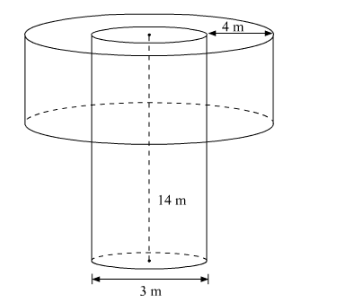# A well of diameter 3 m ad 14 m deep is dug.`
Question:

A well of diameter 3 m ad 14 m deep is dug. The earth, taken out of it, has been evenly spread all around it in the shape of a circular ring of width 4 m to form an embankment. Find the height of the embankment.

Solution:

Given a well with diameter 3 m and height 14 m. The earth dug out from well is used to make a circular embankment of 4m width.

We have to find the height of the embankment.Let be the radius of well

Let be the height of well

Let be the radius of embankment

Let h be the height of embankment

$R=\frac{1}{2} \times$ diameter of well

$=\frac{1}{2} \times 3$

$=1.5 \mathrm{~m}$

$H=14 \mathrm{~m}$

Width of the circular embankment $=4 \mathrm{~m}$

Radius of the circular embankment $r=($ Width of embankment $+R) \mathrm{m}$

$=(4+1.5) \mathrm{m}$

$=5.5 \mathrm{~m}$

Volume of well (Earth Dug) $=\pi R^{2} H$

$=\pi \times\left[(1.5)^{2}\right] \times 14$

Volume of the circular embankment $=\pi r^{2} h$

$=\pi \times\left[(5.5)^{2}-(1.5)^{2}\right] \times h$

According to the question

Volume of the earth dug = Volume of the circular embankment

Therefore,

$\pi \times(1.5)^{2} \times 14=\pi \times\left[(5.5)^{2}-(1.5)^{2}\right] \times h$

$\frac{3}{2} \times \frac{3}{2} \times 14=[(5.5-1.5)(5.5+1.5)] \times h$

$\frac{9}{4} \times 14=4 \times 7 \times h$

$h=\frac{9 \times 14}{4 \times 4 \times 7}$

$h=\frac{9}{8} \mathrm{~m}$